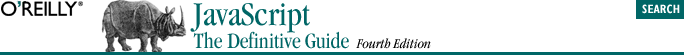home | O'Reilly's CD bookshelfs | FreeBSD | Linux | Cisco | Cisco Exam## 5.3. Arithmetic Operators

The + operator adds numeric operands or concatenates string operands. If one operand is a string, the other is converted to a string and the two strings are then concatenated. Object operands are converted to numbers or strings that can be added or concatenated. The conversion is performed by the valueOf( ) method and/or the toString( ) method of the object.

Subtraction (-)
When - is used as a binary operator, it subtracts its second operand from its first operand. If used with non-numeric operands, it attempts to convert them to numbers.

Multiplication (*)
The * operator multiplies its two operands. If used with non-numeric operands, it attempts to convert them to numbers.

Division (/)
The / operator divides its first operand by its second. If used with non-numeric operands, it attempts to convert them to numbers. If you are used to programming languages that distinguish between integer and floating-point numbers, you might expect to get an integer result when you divide one integer by another. In JavaScript, however, all numbers are floating-point, so all divisions have floating-point results: 5/2 evaluates to 2.5, not 2. Division by zero yields positive or negative infinity, while 0/0 evaluates to NaN.

Modulo (%)
The % operator computes the first operand modulo the second operand. That is, it returns the remainder when the first operand is divided by the second operand an integral number of times. If used with non-numeric operands, the modulo operator attempts to convert them to numbers. The sign of the result is the same as the sign of the first operand. For example, 5 % 2 evaluates to 1.

While the modulo operator is typically used with integer operands, it also works for floating-point values. For example, -4.3 % 2.1 evaluates to -0.1.

Unary minus (-)
When - is used as a unary operator, before a single operand, it performs unary negation. In other words, it converts a positive value to an equivalently negative value, and vice versa. If the operand is not a number, this operator attempts to convert it to one.

Unary plus (+)
For symmetry with the unary minus operator, JavaScript also has a unary plus operator. This operator allows you to explicitly specify the sign of numeric literals, if you feel that this will make your code clearer:

`var profit = +1000000;`

In code like this, the + operator does nothing; it simply evaluates to the value of its argument. Note, however, that for non-numeric arguments, the + operator has the effect of converting the argument to a number. It returns NaN if the argument cannot be converted.

Increment (++)
The ++ operator increments (i.e., adds 1 to) its single operand, which must be a variable, an element of an array, or a property of an object. If the value of this variable, element, or property is not a number, the operator first attempts to convert it to one. The precise behavior of this operator depends on its position relative to the operand. When used before the operand, where it is known as the pre-increment operator, it increments the operand and evaluates to the incremented value of that operand. When used after the operand, where it is known as the post-increment operator, it increments its operand but evaluates to the unincremented value of that operand. If the value to be incremented is not a number, it is converted to one by this process.

For example, the following code sets both i and j to 2:

```i = 1;
j = ++i; ```

But these lines set i to 2 and j to 1:

```i = 1;
j = i++; ```
Decrement (--)
The -- operator decrements (i.e., subtracts 1 from) its single numeric operand, which must be a variable, an element of an array, or a property of an object. If the value of this variable, element, or property is not a number, the operator first attempts to convert it to one. Like the ++ operator, the precise behavior of -- depends on its position relative to the operand. When used before the operand, it decrements and returns the decremented value. When used after the operand, it decrements the operand but returns the undecremented value.